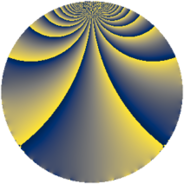# Properties

 Label 588.1.pLevel $588$ Weight $1$ Character orbit 588.p Rep. character $\chi_{588}(557,\cdot)$ Character field $\Q(\zeta_{6})$ Dimension $2$ Newform subspaces $1$ Sturm bound $112$ Trace bound $0$

# Related objects

## Defining parameters

 Level: $$N$$ $$=$$ $$588 = 2^{2} \cdot 3 \cdot 7^{2}$$ Weight: $$k$$ $$=$$ $$1$$ Character orbit: $$[\chi]$$ $$=$$ 588.p (of order $$6$$ and degree $$2$$) Character conductor: $$\operatorname{cond}(\chi)$$ $$=$$ $$21$$ Character field: $$\Q(\zeta_{6})$$ Newform subspaces: $$1$$ Sturm bound: $$112$$ Trace bound: $$0$$

## Dimensions

The following table gives the dimensions of various subspaces of $$M_{1}(588, [\chi])$$.

Total New Old
Modular forms 54 2 52
Cusp forms 6 2 4
Eisenstein series 48 0 48

The following table gives the dimensions of subspaces with specified projective image type.

$$D_n$$ $$A_4$$ $$S_4$$ $$A_5$$
Dimension 2 0 0 0

## Trace form

 $$2 q + q^{3} - q^{9} + O(q^{10})$$ $$2 q + q^{3} - q^{9} + 2 q^{13} - q^{19} - q^{25} - 2 q^{27} - q^{31} + q^{37} + q^{39} - 2 q^{43} - 2 q^{57} + 2 q^{61} + q^{67} - q^{73} + q^{75} + q^{79} - q^{81} + q^{93} - 4 q^{97} + O(q^{100})$$

## Decomposition of $$S_{1}^{\mathrm{new}}(588, [\chi])$$ into newform subspaces

Label Dim $A$ Field Image CM RM Traces $q$-expansion
$a_{2}$ $a_{3}$ $a_{5}$ $a_{7}$
588.1.p.a $2$ $0.293$ $$\Q(\sqrt{-3})$$ $D_{3}$ $$\Q(\sqrt{-3})$$ None $$0$$ $$1$$ $$0$$ $$0$$ $$q-\zeta_{6}^{2}q^{3}-\zeta_{6}q^{9}+q^{13}-\zeta_{6}q^{19}+\cdots$$

## Decomposition of $$S_{1}^{\mathrm{old}}(588, [\chi])$$ into lower level spaces

$$S_{1}^{\mathrm{old}}(588, [\chi]) \cong$$ $$S_{1}^{\mathrm{new}}(84, [\chi])$$$$^{\oplus 2}$$